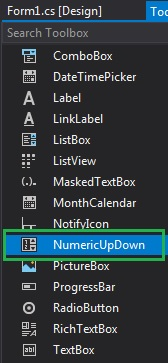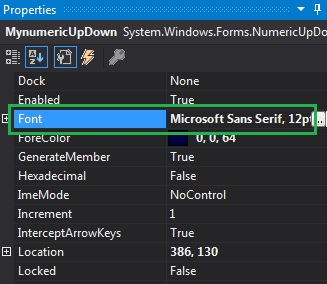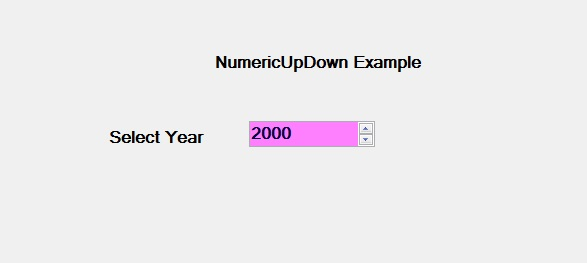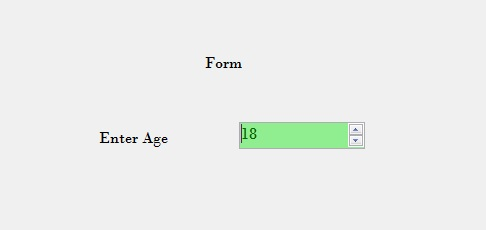# How to set Font of the NumericUpDown in C#?

In Windows Forms, NumericUpDown control is used to provide a Windows spin box or an up-down control which displays the numeric values. Or in other words, NumericUpDown control provides an interface which moves using up and down arrow and holds some pre-defined numeric value. In NumericUpDown control, you can set the font of the content present in the up-down control using the Font Property. You can set this property in two different ways:

1. Design-Time: It is the easiest way to set the font of the NumericUpDown as shown in the following steps:

• Step 1: Create a windows form as shown in the below image:

Visual Studio -> File -> New -> Project -> WindowsFormApp• Step 2: Next, drag and drop the NumericUpDown control from the toolbox on the form as shown in the below image:• Step 3: After drag and drop you will go to the properties of the NumericUpDown and set the font of the NumericUpDown as shown in the below image;Output:2. Run-Time: It is a little bit trickier than the above method. In this method, you can set the font of the content present in the NumericUpDown control programmatically with the help of given syntax:

`public virtual System.Drawing.Font Font { get; set; }`

Here, Font represents the font of the content present in the NumericUpDown control. The following steps show how to set the font of the NumericUpDown dynamically:

• Step 1: Create a NumericUpDown using the NumericUpDown() constructor is provided by the NumericUpDown class.
```// Creating a NumericUpDown
NumericUpDown n = new NumericUpDown();
```
• Step 2: After creating NumericUpDown, set the Font property of the NumericUpDown provided by the NumericUpDown class.
```// Setting the font
n.Font = new Font("Bodoni MT", 12);
```
• Step 3: And last add this NumericUpDown control to the form using the following statement:
```// Adding NumericUpDown control on the form
```

Example:

 `using` `System; ` `using` `System.Collections.Generic; ` `using` `System.ComponentModel; ` `using` `System.Data; ` `using` `System.Drawing; ` `using` `System.Linq; ` `using` `System.Text; ` `using` `System.Threading.Tasks; ` `using` `System.Windows.Forms; ` ` `  `namespace` `WindowsFormsApp42 { ` ` `  `public` `partial` `class` `Form1 : Form { ` ` `  `    ``public` `Form1() ` `    ``{ ` `        ``InitializeComponent(); ` `    ``} ` ` `  `    ``private` `void` `Form1_Load(``object` `sender, EventArgs e) ` `    ``{ ` ` `  `        ``// Creating and setting the  ` `        ``// properties of the labels ` `        ``Label l1 = ``new` `Label(); ` `        ``l1.Location = ``new` `Point(348, 61); ` `        ``l1.Size = ``new` `Size(215, 20); ` `        ``l1.Text = ``"Form"``; ` `        ``l1.Font = ``new` `Font(``"Bodoni MT"``, 12); ` `        ``this``.Controls.Add(l1); ` ` `  `        ``Label l2 = ``new` `Label(); ` `        ``l2.Location = ``new` `Point(242, 136); ` `        ``l2.Size = ``new` `Size(103, 20); ` `        ``l2.Text = ``"Enter Age"``; ` `        ``l2.Font = ``new` `Font(``"Bodoni MT"``, 12); ` `        ``this``.Controls.Add(l2); ` ` `  `        ``// Creating and setting the  ` `        ``// properties of NumericUpDown ` `        ``NumericUpDown n = ``new` `NumericUpDown(); ` `        ``n.Location = ``new` `Point(386, 130); ` `        ``n.Size = ``new` `Size(126, 26); ` `        ``n.Font = ``new` `Font(``"Bodoni MT"``, 12); ` `        ``n.Value = 18; ` `        ``n.Minimum = 18; ` `        ``n.Maximum = 30; ` `        ``n.BackColor = Color.LightGreen; ` `        ``n.ForeColor = Color.DarkGreen; ` `        ``n.Increment = 1; ` `        ``n.Name = ``"MySpinBox"``; ` ` `  `        ``// Adding this control  ` `        ``// to the form ` `        ``this``.Controls.Add(n); ` `    ``} ` `} ` `} `

Output:My Personal Notes arrow_drop_upCheck out this Author's contributed articles.

If you like GeeksforGeeks and would like to contribute, you can also write an article using contribute.geeksforgeeks.org or mail your article to contribute@geeksforgeeks.org. See your article appearing on the GeeksforGeeks main page and help other Geeks.

Please Improve this article if you find anything incorrect by clicking on the "Improve Article" button below.

Article Tags :

Be the First to upvote.

Please write to us at contribute@geeksforgeeks.org to report any issue with the above content.